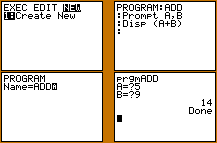Go to contentHow to write a simple program to add two numbers together on a GDC (graphical display calculator). This explanation uses the TI-82/83/84 calculators.

1. Press PRGM to select the program entry menu.
2. Select NEW, enter the name "ADD" and begin entering the program line by line.
3. Once the program is completed, quit then select PRGM again and the program name "ADD".

You will be prompted for the values of A and B, then the sum will be displayed.You can get a better display of the maths by downloading special TeX fonts from jsMath. In the meantime, we will do the best we can with the fonts you have, but it may not be pretty and some equations may not be rendered correctly.

## Glossary

### gdc

graphic display calculator

### union

The union of two sets A and B is the set containing all the elements of A and B.

Full Glossary List

## This question appears in the following syllabi:

SyllabusModuleSectionTopicExam Year
AQA A-Level (UK - Pre-2017)D1AlgorithmsCode-
AQA A2 Further Maths 2017Discrete MathsAlgorithms and Graph Theory - ExtraAlgorithms-
AQA AS/A2 Further Maths 2017Discrete MathsAlgorithms and Graph Theory - ExtraAlgorithms-
Edexcel A-Level (UK - Pre-2017)D1AlgorithmsCode-
Edexcel AS Further Maths 2017Decision Maths 1Algorithms and Graph TheoryAlgorithms-
Edexcel AS/A2 Further Maths 2017Decision Maths 1Algorithms and Graph TheoryAlgorithms-
OCR A-Level (UK - Pre-2017)D1AlgorithmsCode-
OCR AS Further Maths 2017Discrete MathsAlgorithmsAlgorithms-
OCR MEI AS Further Maths 2017Modelling with AlgorithmsAlgorithmsAlgorithms-
OCR-MEI A-Level (UK - Pre-2017)D1AlgorithmsCode-
Universal (all site questions)AAlgorithmsCode-